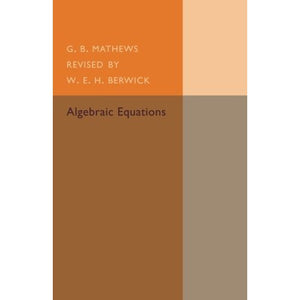># Algebraic Equations (Cambridge Tracts in Mathematics)

• £7.79
• Save £7.20

G. B. Mathews
Cambridge University Press, 3/26/2015
EAN 9781107493612, ISBN10: 1107493617

Paperback, 78 pages, 21.6 x 14 x 0.5 cm
Language: English

First published in 1930, as the third edition of a 1907 original, this book forms number six in the Cambridge Tracts in Mathematics and Mathematical Physics Series. The text gives a concise account of the theory of equations according to the ideas of Galois. This book will be of value to anyone with an interest in algebra and the history of mathematics.

1. Galosian groups and resolvents
2. Cyclical equations
3. Abelian equations
4. Metacyclic equations
quintic and sextic equations
5. Solution by standard forms
Notes and references.